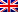# Johnson's algorithm

Contenus

## Johnson's algorithm

Johnson's algorithm calculates the shortest paths for any pair of vertices in the graph. He has a good complexity for sparse graphs (few edges compared to the number of vertices).

It uses the algorithms of Dijkstra and of Bellman Ford, and returns either the matrix of shortest path weights or the assertion that the graph has a negative weight path.
The technique used consists in coming back to the case with only positive weights, then to run the Dijkstra algorithm starting from each vertex. If the weights are not all positive, we redefine them to be able to reduce to the first case.

Johnson's algorithm is made up of the following steps:

1. Add a new node q to the graph, and connect this node by arcs of zero weight to all the other nodes.
2. Use the Bellman-Ford algorithm starting from the new node q to determine, for each vertex v, the minimum weight h(v) from a path of q To v. If a negative cycle is detected, the algorithm stops on failure.
3. Respond the arcs of the initial graph using the values calculated by the Bellman-Ford algorithm: an arc of u To v, whose former weight or length is the number w (u, v), has for new length the non-negative number w (u, v) + h (u) - H v).
4. Remove the top q and use Dijkstra's algorithm to determine the shortest paths.

Example of Johnson's algorithm:FRFRENES